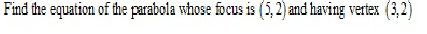# RD Sharma Solution CLass 11 Mathematics Chapter 25 Parabola

## Chapter 25 – Parabola Exercise Ex. 25.1

Question 1Solution 1Question 2Solution 2Question 3Solution 3Question 4Solution 4Question 5Solution 5Question 6Solution 6Question 7Solution 7Question 8Solution 8Question 9Solution 9Question 10Solution 10Question 11Solution 11Question 12Solution 12Question 13Solution 13Question 14Solution 14Question 15Solution 15Question 16Solution 16Question 17Solution 17Question 18Solution 18Question 19Solution 19Question 20Solution 20Question 21

Find the area of the triangle formed by the lines joining the vertex of the parabolato the ends of its latus- rectum

Solution 21Question 22Solution 22Question 23Solution 23Question 24Solution 24Question 25Solution 25Question 26Solution 26Question 27Solution 27Question 28

Find the equation of the lines joining the vertex of the parabola y2 = 6x to the point on it which have abscissa 24

Solution 28Question 29

Find the coordinates of points on the parabola y2 = 8x whose focal distance is 4

Solution 29

In given parabola

a=2

Given focal distance=a+x=4, so x=2

So points are (2, 4) and (2, -4)

Question 30

Find the length  of the lines segment joining the vertex of the parabola y2 = 4ax and a point on the parabola where the line-segment makes an angleto the axis

Solution 30Question 31

If the points (0, 4) and (0, 2) are respectively the
vertex and focus of a parabola, then find the equation of the parabola.

Solution 31Question 32

If the line y = mx + 1 is tangent to the parabola y2 = 4x,
then find the value of m.

Solution 32## Chapter 25 – Parabola Exercise Ex. 25VSAQ

Question 1Solution 1Question 2Solution 2Question 3Solution 3Question 4Solution 4Question 5Solution 5Question 6Solution 6Question 7Solution 7Question 8Solution 8Question 9Solution 9error: Content is protected !!